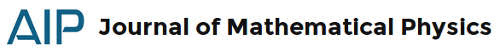### JMP 58, 3: math paradoxes in quantum mechanicsFacchi, P., & Ligabò, M. (2017). Large-time limit of the quantum Zeno effect Journal of Mathematical Physics, 58 (3) DOI: 10.1063/1.4978851 (arXiv)
If very frequent periodic measurements ascertain whether a quantum system is still in its initial state, its evolution is hindered. This peculiar phenomenon is called quantum Zeno effect. We investigate the large-time limit of the survival probability as the total observation time scales as a power of the measurement frequency, $t \propto N^\alpha$. The limit survival probability exhibits a sudden jump from $1$ to $0$ at $\alpha = 1/2$, the threshold between the quantum Zeno effect and a diffusive behavior. Moreover, we show that for $\alpha \geq 1$, the limit probability becomes sensitive to the spectral properties of the initial state and to the arithmetic properties of the measurement periods.
Selvitella, A. (2017). The Simpson’s paradox in quantum mechanics Journal of Mathematical Physics, 58 (3) DOI: 10.1063/1.4977784 (sci-hub)
In probability and statistics, the Simpson’s paradox is a paradox in which a trend that appears in different groups of data disappears when these groups are combined, while the reverse trend appears for the aggregate data. In this paper, we give some results about the occurrence of the Simpson’s paradox in quantum mechanics. In particular, we prove that the Simpson’s paradox occurs for solutions of the quantum harmonic oscillator both in the stationary case and in the non-stationary case. In the non-stationary case, the Simpson’s paradox is persistent: if it occurs at any time $t=\tilde t$, then it occurs at any time $t\not= \tilde t$. Moreover, we prove that the Simpson’s paradox is not an isolated phenomenon, namely, that, close to initial data for which it occurs, there are lots of initial data (a open neighborhood), for which it still occurs. Differently from the case of the quantum harmonic oscillator, we also prove that the paradox appears (asymptotically) in the context of the nonlinear Schrödinger equation but at intermittent times.
Meng, F., & Liu, C. (2017). Necessary and sufficient conditions for the existence of time-dependent global attractor and application Journal of Mathematical Physics, 58 (3) DOI: 10.1063/1.4978329 (sci-hub)
In this paper, we are concerned with infinite dimensional dynamical systems in time-dependent space. First, we characterize some necessary and sufficient conditions for the existence of the time-dependent global attractor by using a measure of noncompactness. Then, we give a new method to verify the sufficient condition. As a simple application, we prove the existence of the time-dependent global attractor for the damped equation in strong topological space.
Cen, J., Correa, F., & Fring, A. (2017). Time-delay and reality conditions for complex solitons Journal of Mathematical Physics, 58 (3) DOI: 10.1063/1.4978864 (arXiv)
We compute lateral displacements and time-delays for scattering processes of complex multi-soliton solutions of the Korteweg de-Vries equation. The resulting expressions are employed to explain the precise distinction between solutions obtained from different techniques, Hirota’s direct method and a superposition principle based on Bäcklund transformations. Moreover they explain the internal structures of degenerate compound multi-solitons previously constructed. Their individual one-soliton constituents are time-delayed when scattered amongst each other. We present generic formulae for these time-dependent displacements. By recalling Gardner’s transformation method for conserved charges, we argue that the structure of the asymptotic behaviour resulting from the integrability of the model together with its $PT$-symmetry ensures the reality of all of these charges, including in particular the mass, the momentum, and the energy.
Wilming, H., Kastoryano, M., Werner, A., & Eisert, J. (2017). Emergence of spontaneous symmetry breaking in dissipative lattice systems Journal of Mathematical Physics, 58 (3) DOI: 10.1063/1.4978328 (arXiv)
A cornerstone of the theory of phase transitions is the observation that many-body systems exhibiting a spontaneous symmetry breaking in the thermodynamic limit generally show extensive fluctuations of an order parameter in large but finite systems. In this work, we introduce the dynamical analog of such a theory. Specifically, we consider local dissipative dynamics preparing an equilibrium steady-state of quantum spins on a lattice exhibiting a discrete or continuous symmetry but with extensive fluctuations in a local order parameter. We show that for all such processes, there exist asymptotically stationary symmetry-breaking states, i.e., states that become stationary in the thermodynamic limit and give a finite value to the order parameter. We give results both for discrete and continuous symmetries and explicitly show how to construct the symmetry-breaking states. Our results show in a simple way that, in large systems, local dissipative dynamics satisfying detailed balance cannot uniquely and efficiently prepare states with extensive fluctuations with respect to local operators. We discuss the implications of our results for quantum simulators and dissipative state preparation.